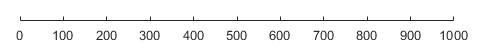Documentation

# uiaxes

Create UI axes for plots in App Designer

## Syntax

``ax = uiaxes``
``ax = uiaxes(Name,Value)``
``ax = uiaxes(parent)``
``ax = uiaxes(parent,Name,Value)``

## Description

example

````ax = uiaxes` creates UI axes in a new figure window and returns the `UIAxes` object. MATLAB® calls the `uifigure` function to create the figure.```

example

````ax = uiaxes(Name,Value)` specifies `UIAxes` property values using one or more `Name,Value` pair arguments.```

example

````ax = uiaxes(parent)` creates the UI axes in the specified parent container. The parent can be a `Figure` created using the `uifigure` function, or one of its child containers.```
````ax = uiaxes(parent,Name,Value)` specifies `UIAxes` property values using one or more `Name,Value` arguments.```

## Examples

collapse all

Create a line plot and a scatter plot in UI axes.

Create a figure window with UI axes and assign the `UIAxes` object to the variable `ax`. Add a line plot to the axes by specifying the `UIAxes` object as the first input argument for the `plot` function.

```fig = uifigure; ax = uiaxes(fig); x = linspace(-pi,pi,50); y = 5*sin(x); plot(ax,x,y) ```Set the hold state on and add a scatter plot. Specify the `UIAxes` object as the first input argument for the `hold` and `scatter` functions.

```hold(ax,'on') y2 = 5*sin(x) + randn(1,50); scatter(ax,x,y2)```Modify the appearance of the UI axes by setting properties using name-value pair arguments. For example, reverse the x-axis direction using the `XDir` name-value pair.

```fig = uifigure; ax = uiaxes(fig,'XDir','reverse'); x = linspace(-pi,pi); y = sin(x); plot(ax,x,y)```Alternatively, specify properties after the axes is created using dot notation. For example, reverse the y-axis direction using dot notation to access the `YDir` property.

`ax.YDir = 'reverse';`

Specify the UI axes position by setting the `Position` property. Specify the position in pixels.

```fig = uifigure; ax = uiaxes(fig,'Position',[10 10 550 400]); ```Add UI axes to a panel within a figure window. Specify the panel and axes positions in pixels.

```fig = uifigure; p = uipanel(fig,'Position',[10 10 400 400]); ax = uiaxes(p,'Position',[10 10 390 390]);```## Input Arguments

collapse all

Parent container, specified as a `Figure` object created using the `uifigure` function, or one of its child containers: `Tab`, `Panel`, `ButtonGroup`, or `GridLayout`. If you do not specify a parent container, MATLAB calls the `uifigure` function to create a new `Figure` object that serves as the parent container.

### Name-Value Pair Arguments

Specify optional comma-separated pairs of `Name,Value` arguments. `Name` is the argument name and `Value` is the corresponding value. `Name` must appear inside quotes. You can specify several name and value pair arguments in any order as `Name1,Value1,...,NameN,ValueN`.

Example: `'Xscale','linear','YScale','log'`

The properties list here are only a subset. For a full list, see UIAxes Properties.

Minimum and maximum limits, specified as a two-element vector of the form `[min max]`, where `max` is greater than `min`. You can specify the limits as numeric, categorical, datetime, or duration values. However, the type of values that you specify must match the type of values along the axis.

You can specify both limits or you can specify one limit and let the axes automatically calculate the other. For an automatically calculated minimum or maximum limit, use `-inf` or `inf`, respectively.

Example: `ax.XLim = [0 10]`

Example: `ax.YLim = [-inf 10]`

Example: `ax.ZLim = [0 inf]`

Alternatively, use the `xlim`, `ylim`, and `zlim` functions to set the limits. For an example, see Specify Axis Limits.

Data Types: `single` | `double` | `int8` | `int16` | `int32` | `int64` | `uint8` | `uint16` | `uint32` | `uint64` | `datetime` | `duration`

Axis scale, specified as one of these values.

ValueDescriptionResult
`'linear'`

Linear scale

Example: `ax.XScale = 'linear'``'log'`

Log scale

Example: `ax.XScale = 'log'`Line style for grid lines, specified as one of the line styles in this table.

Line StyleDescriptionResulting Line
`'-'`Solid line`'--'`Dashed line`':'`Dotted line`'-.'`Dash-dotted line`'none'`No lineNo line

To display the grid lines, use the `grid on` command or set the `XGrid`, `YGrid`, or `ZGrid` property to `'on'`.

Example: `ax.GridLineStyle = '--'`

Size and location of axes, including the labels and margins, specified as a four-element vector of the form ```[left bottom width height]```. This vector defines a rectangle that encloses the outer bounds of the axes. The `left` and `bottom` elements define the position of the rectangle, measured from the lower left corner to the lower left corner of the parent container. The `width` and `height` define the size of the rectangle. The values are measured in units determined by the `Units` property. By default, the units are pixels.

## Output Arguments

collapse all

`UIAxes` object. Use `ax` to set properties of the `UIAxes` after they are created.

## Tips

• Zoom, pan, and rotate modes only support a subset of options for UI axes. `zoom` only supports the `on`, `off`, and zoom factor arguments. `rotate3d` and `pan` only support the `on` and `off` arguments. The recommended way to interact with charts in the UI axes is to use the axes toolbar or the built-in axes interactions. For more information, see Control Chart Interactivity.